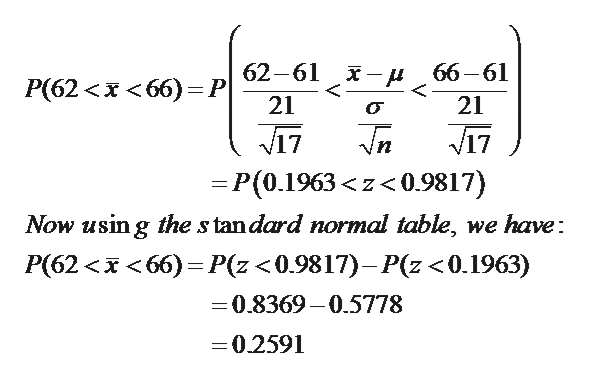# The average production cost for major movies is 61 million dollars and the standard deviation is 21 million dollars. Assume the production cost distribution is normal. Suppose that 17 randomly selected major movies are researched. Answer the following questions. Round all answers to 4 decimal places where possible.For the group of 17 movies, find the probability that the average production cost is between 62 and 66 million dollars. For part d), is the assumption of normal necessary? YesNo

Question
37 views

The average production cost for major movies is 61 million dollars and the standard deviation is 21 million dollars. Assume the production cost distribution is normal. Suppose that 17 randomly selected major movies are researched. Answer the following questions. Round all answers to 4 decimal places where possible.

1. For the group of 17 movies, find the probability that the average production cost is between 62 and 66 million dollars.
2. For part d), is the assumption of normal necessary? YesNo
check_circle

Step 1

Solution: We are given that the average production cost for major movies is 61 million dollars and the standard deviation is 21 million dollars. The production cost distribution is assumed to be normal.

Step 2

We have to find for the group of 17 movies, the probability that the average production cost is between 62 and 66 million dollars

Step 3

Using the z-score formula, w...help_outlineImage Transcriptionclose62-61 х— и 66—61 Р(62 <х <66) 3DP 21 21 о 17 = P (0.1963

### Want to see the full answer?

See Solution

#### Want to see this answer and more?

Solutions are written by subject experts who are available 24/7. Questions are typically answered within 1 hour.*

See Solution
*Response times may vary by subject and question.
Tagged in

### Measures